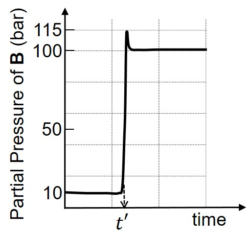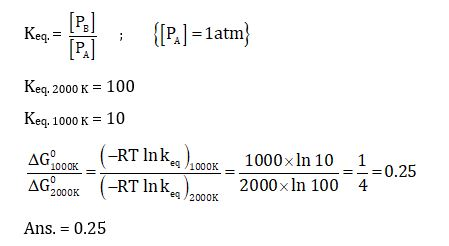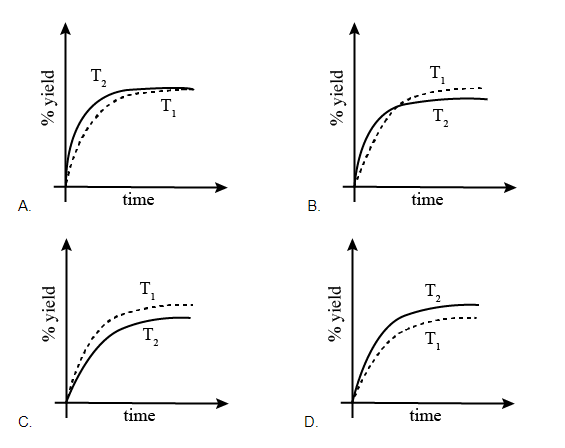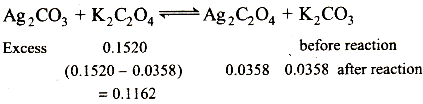Win up to 100% scholarship on Aakash BYJU'S JEE/NEET courses with ABNAT Win up to 100% scholarship on Aakash BYJU'S JEE/NEET courses with ABNAT

# Chemical Equilibrium ‌–‌ ‌JEE‌ ‌Advanced‌ ‌Previous‌ ‌Year‌ ‌Questions with Solutions

Chemical Equilibrium JEE Advanced Previous Year Questions with Solutions that are provided here consists of a set of questions that appeared in the various past sessions of the entrance exam. In order to help JEE aspirants prepare well and score maximum marks in the JEE Advanced this set of questions and solutions will play an important role in helping them achieve this goal. As aspirants go through the questions and solutions they will further get familiar with the format of the question paper, its types and the difficulty level. Additionally, as JEE aspirants engage in constant practice to solve all the questions they will acquire adept problem-solving skills to tackle the different types of questions that could appear in the upcoming exam.

Students or JEE aspirants likewise can also download Chemical Equilibrium JEE Advanced Previous Year Questions with Solutions directly from here in the form of a PDF.

## JEE Advanced Previous Year Questions on Chemical Equilibrium

Question 1. Consider the reaction A ⇌ B at 1000 K. At time t’, the temperature of the system was increased to 2000 K and the system was allowed to reach equilibrium. Throughout this experiment, the partial pressure of A was maintained at 1 bar. Given below is the plot of the partial pressure of B with time. What is the ratio of the standard Gibbs energy of the reaction at 1000 K to that at 2000 K?Solution: (0.25)Question 2. For the following reaction, the equilibrium constant Kc at 298 K is 1.6 × 1017.

Fe(aq)2+ + S(aq)2- ⇌ FeS(s)

When equal volumes of 0.06 M Fe(aq)2+ and 0.2 M S(aq)2- solutions are mixed, the equilibrium concentration of Fe2+(aq) is found to be Y × 10-17 M. The value of Y is.

Solution: (8.93)

Ini 0.06 0.2 0
After mix 0.03 0.1
At 0 q/m x 0.07

Kc = 1 / (x) [0.07] = 1.6 × 1017

∴ [Fe2+] = 8.928 × 10-17

= y × 10-17

∴ y = 8.93 or 8.92

Question 3. The % yield of ammonia as a function of time in the reaction:

N2(g) + 3H2(g) ⇌ 2NH3(g), ΔH < 0 at (P, T1) is given below.If this reaction is conducted at (P, T2), with T2 > T1, the %yield of ammonia as a function of time is represented by:Solution: (B)

Initially on increasing temperature, the rate of reaction increases, so % yield also increases with time. But at equilibrium % yield at high temperature (T2) would be less than at T1 as the reaction is exothermic so the graph isQuestion 4. The thermal dissociation of equilibrium of CaCO3 (s) is studied under different conditions: CaCO3(g) ⇌ CaCO(g) + CO2(g)

For this equilibrium, the correct statement(s) is/are

A. △ H is dependent on T

B. K is independent of the initial amount of CaCO3

C. K is dependent on the pressure of CO2 at a given T

D. △ H is independent of the catalyst, if any

Solution: (A, B and D)

A. ΔH = Cp(rxn) ΔT

Hence, enthalpy depends upon temperature

B. CaCO3(s) ⇌ CaO(s) + CO2(g) Kp = PcO2

C. Keq, depends only on temperature and not on Pressure.

D. Enthalpy of reaction is independent of the catalyst. Catalyst generally changes activation energy.

Question 5. Solubility product constant (Ksp) of salts of type MX, MX2 and M3X at temperature ‘T’ are 4.0 x 10-8, 3.2 x 10-14 and 2.7 x 10-15, respectively. Solubilities (mol, dm) of the salt at temperature ‘T’ are in the order

A. MX > MX2 > M3X

B. M3X > MX2 > MX

C. MX2 > M3X > MX

D. MX > M3X > MX2

Solution: (D)

MX: Ksp = S2 = 4 × 10-8

⇒ S = 2 × 10-4

MX2: Ksp = 4S3 = 3.2 × 10-14 ⇒ S = 2 × 10-5

M3X: Ksp = 27S4 = 2.7 × 10-15 ⇒ S = 10-4

Order of solubility is MX > M3X > MX2

Question 6. In one liter saturated solution of AgCl [Ksp = 1.6 x 10-10], 0.1 mol of CuCl [Ksp = 1.0 x 10-10 ] is added. The resultant concentration of Ag+ in the solution is 1.6 × 10-x. The value of ‘x’ is ___.

Solution: (7)

It is a case of simultaneous solubility of salts with a common ion. Here, the solubility product of CuCl is much greater than that of AgCl, it can be assumed that Cl in solution comes mainly from CuCl.

[Cl] = √Ksp(CuCl) = 10-3 M

Now, for AgCl, Ksp = 1.6 × 10-10 = [Ag+][Cl]

=[Ag+] × 10-3

⇒ [Ag+] = 1.6 × 10-7

Hence, x = 7

Question 7. Each question contains STATEMENT-1 (Assertion) and STATEMENT-2 (Reason). Examine the statement carefully and mark the correct answer according to the instructions given below:

Statement 1: For every chemical reaction at equilibrium, the standard Gibbs energy of the reaction is zero.

Statement 2: At constant temperature and pressure, chemical reactions are spontaneous in the direction of decreasing Gibbs energy.

A. If both (1) and (2) are correct, and (2) is the correct explanation for (1)

B. If both (1) and (2) are correct, but (2) is not the correct explanation for (1)

C. If (1) is correct, but (2) is incorrect

D. IF (1) is incorrect, but (2) is correct

Solution: (D)

The standard Gibb’s energy for a reaction is given by ΔGo.

At equilibrium, ΔG = 0 whereas ΔGo for a reaction may or may not be zero.

For a spontaneous process, Gibb’s energy for a reaction is always negative, ΔG < 0

Hence, if (1) is incorrect, but (2) is correct

Question 8. The solubility product of Ag2C2O4 at 25°C is 1.29 x 10-11 mol3L-3. A solution of K2C2O4 containing 0.520 moles in 500mL water is shaken at 25°C with an excess of Ag2CO3 till the following equilibrium is reached;

Ag2CO3 + K2C2O4 ⇋ Ag2C2O4 + K2CO3

At equilibrium, the solution contains 0.0358 moles of K2CO3. Assuming the degree of dissociation of K2C2O4 and K2CO3 to be equal, calculate the solubility product of Ag2CO3.

Solution: (3.974 × 10-12 )Initially, the concentration of oxalate ion was 0.1520 moles.

And at equilibrium the amount of carbonate ion formed = 0.1520 − 0.0358 = 0.1162

The concentration of potassium oxalate remaining after the reaction is 0.1162 / 0.5 = 0.2324M.

The concentration of potassium carbonate (or carbonate ions) at equilibrium = 0.0358 / 0.5 = 0.0716M.

For silver oxalate, Ksp = [Ag+]2[C2O42-].

Hence, 1.29 × 10-11 = [Ag+]2 × 0.2324.

[Ag+]2 = 1.29 × 10-11 / 0.2324.

The expression for the solubility product of silver carbonate is

Ksp = [Ag+]2 [CO32-] = 1.29×10-11 / 0.2324 × 0.0716 = 3.974 × 10-12 mol3.lit-3.

Question 9. A sample of AgCl was treated with 10 mL of 1.7 M Na2CO3 solution to give Ag2CO3. The remaining solution contained 0.0026 g of Cl0 per litre. Calculate the solubility product of AgCl. Ksp(Ag2CO3) = 8.2 × 1012.

Solution: (1.71 × 10-10)

The double displacement reaction is:

2AgCl + Na2CO3 → Ag2CO3 + 2NaCl

Ksp(Ag2CO3) = 8.2 × 10-12 (given) = [Ag+]2[CO32-]

Sodium Carbonate will completely dissociate into Na+ and CO32- ions. [CO32-] = [Na2CO3] = 1.5M

From this , we can arrive at [Ag+] = (Ksp(Ag2CO3)[CO32-])0.5 = 2.34 × 10-6 M

Amount of Cl remaining = 00026 g = 0.002635.5 molL−1 = 7.33 × 10-5 M

We need to figure out Ksp (AgCl) = [Ag+][Cl] = 2.34 × 10-6 M × 7.33 × 10-5 M = 1.71 × 10-10

Question 10. For the reaction; PCl5(g) ⇋ PCl3(g) + Cl2(g) The forward reaction at constant temperature is favoured by:

A. Introducing an inert gas at constant volume

B. Introducing chlorine gas at constant volume

C. Introducing an inert gas at constant pressure

D. Increasing the volume of the container

E. Introducing PCl5 at constant volume

Solution: (C, D and E)

C. Adding inert gas at constant pressure increases the overall volume, reduces the concentration of all reactants, changing the partial pressure. The system tries to undo that change and to increase the concentration goes in the direction where moles increase, which is the forward direction in this case.

D. This has a similar effect as adding an inert gas at constant volume. The concentration decreases, decreasing the partial pressure, the reaction moves forward to increase the number of moles.

E. Also adding PCI5 (g )at constant volume will favour forward reaction because PCI5 (g ) is a reactant.

## Related Videos

### Introduction To Chemical Equilibrium### Chemical Equilibrium